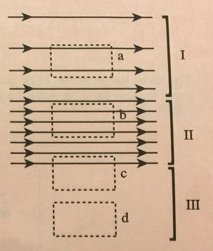# Problem: A current distribution (not shown) creates the spatially varying magnetic field shown at right. In region I, the magnetic field increases in magnitude toward the bottom of the page. In region II, the magnetic field is uniform. In region III, the magnetic field is zero. a. Consider loop a (in region I).i. Draw and label an area vector for loop a on the diagram. ii. In which direction should you integrate around the loop? Explain your reasoning. iii. Is the line integral of the magnetic field around loop a positive, negative, or zero? Explain your reasoning. iv. In which direction is the net current passing through the loop? Use Ampere's law to explain your answer. If no net current passes through the loop, state so explicitly. b. In which is the net current passing through loop b (in region I Explain, using the reasoning you applied to loop a.c. In which direction is the net current passing through loop c (the top part of which is in region II, and the bottom part of which is in region III)? Explain, using the reasoning you applied to loop a.d. In which direction is the net current passing through loop d (in region III)? Explain, using the reasoning you applied to loop a.

###### FREE Expert Solution

a. i.

The area vector is always directed perpendicularly outward from the area.

95% (167 ratings)###### Problem Details

A current distribution (not shown) creates the spatially varying magnetic field shown at right. In region I, the magnetic field increases in magnitude toward the bottom of the page. In region II, the magnetic field is uniform. In region III, the magnetic field is zero.a. Consider loop a (in region I).

i. Draw and label an area vector for loop a on the diagram.

ii. In which direction should you integrate around the loop? Explain your reasoning.

iii. Is the line integral of the magnetic field around loop a positive, negative, or zero? Explain your reasoning.

iv. In which direction is the net current passing through the loop? Use Ampere's law to explain your answer. If no net current passes through the loop, state so explicitly.

b. In which is the net current passing through loop b (in region I Explain, using the reasoning you applied to loop a.

c. In which direction is the net current passing through loop c (the top part of which is in region II, and the bottom part of which is in region III)? Explain, using the reasoning you applied to loop a.

d. In which direction is the net current passing through loop d (in region III)? Explain, using the reasoning you applied to loop a.